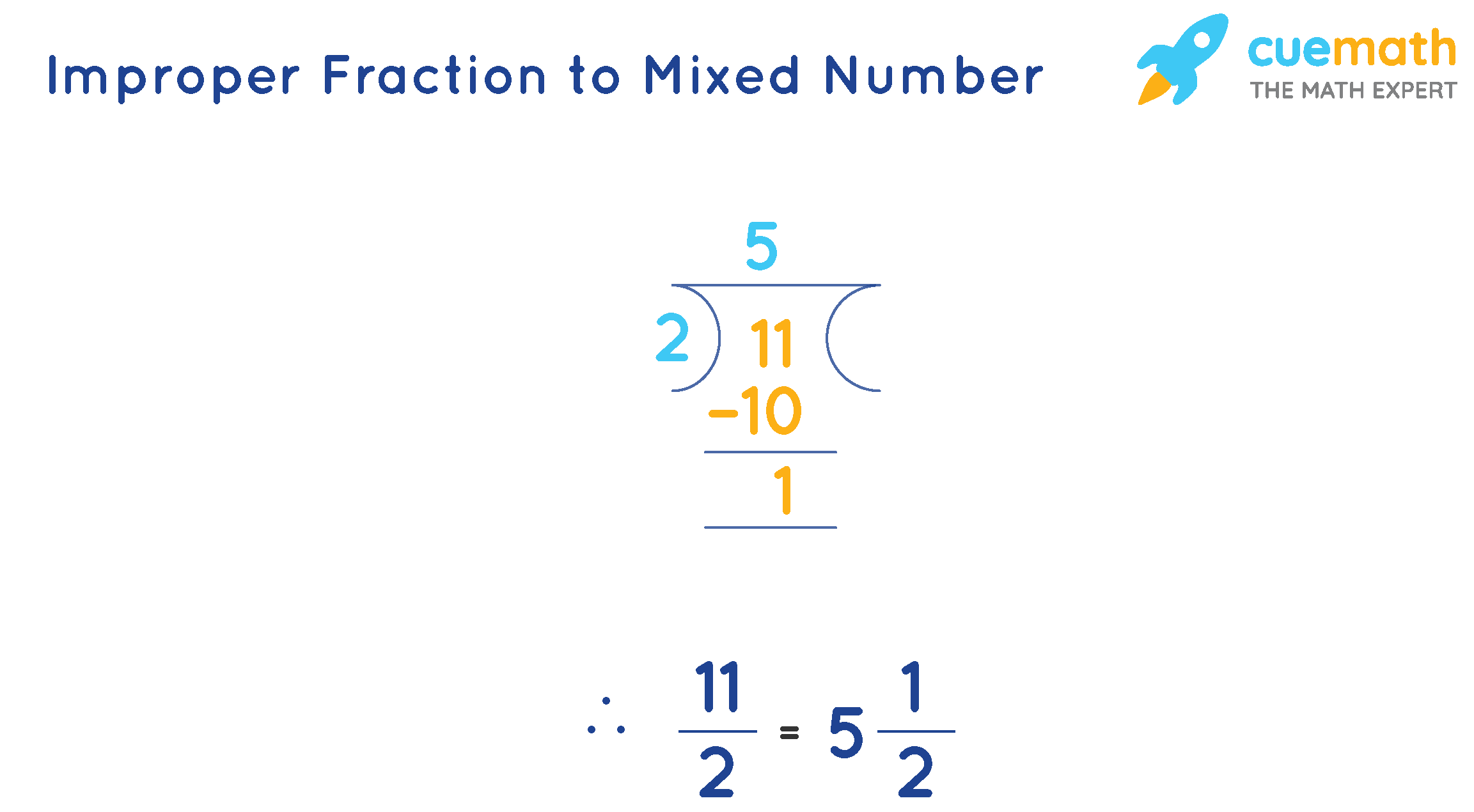# Decimal to Mixed Number Calculator

## What Is a Decimal to Mixed Number Calculator?

A Decimal to Mixed Number Calculator is a free online tool which converts decimal numbers to mixed fractions. Cuemath's online calculator helps you to calculate faster and displays the result within a few seconds.

Note: Enter numbers up to 4 digits

## How to Use the Decimal to Mixed Number Calculator?

Follow the steps given below to use the calculator.

• Step 1: Enter the decimal number in the input box.
• Step 2: Click on "Calculate" to get the mixed fraction for the decimal number that was entered.
• Step 3: Click on "Reset" to clear the fields and enter the new decimal number.

## How to Convert a Decimal Number to a Mixed Fraction?

A decimal number can be converted to a mixed fraction in the following way:

• Convert the decimal number to an improper fraction.
• Now, convert the improper fraction to a mixed number by dividing the numerator by the denominator.

### Solved Example:

Convert 5.5 to a mixed fraction.

### Solution:

There is only one digit after the decimal, so 5.5 can be written as 55/10. This can be further simplified to 11/2.

Now, divide 11 by 2.Therefore, 5.5, in the mixed fraction form is  $$5\frac{1}{2}$$

Now, try to use this calculator and convert the following decimal numbers to mixed fractions:

• 6.75
• 0.8
• 3.12